# Internal vs. External Volume Control

#93 in a series of articles about the technology behind Bang & Olufsen

A question came to my desk this week from a customer who would like to connect a third-party streaming device to his Beolab 50s. He plans to use a USB-Audio connection and his question was “Should I control the volume of the audio signal in the streamer or in the Beolab 50s?” There are three different ways to configure these two options:

1. Control the volume in the streamer using its interface, and send a signal that has been volume-regulated to the Beolab 50s, which should then be set to have a start up default volume such that the maximum volume on the streamer results in a level that is as loud as the customer will ever want it to be. In order to do this, the Beolab 50s need to be set to ignore the volume information that is received on the USB-Audio connection.
2. Set the streamer to output an unregulated signal, and set the Beolab 50s to obey the volume information that is received on the USB-Audio connection, then use the streamer’s interface for the volume control (which would actually be happening inside the Beolab 50s).
3. Set the streamer to output an unregulated signal, and set the Beolab 50s to disobey the volume information that is received on the USB-Audio connection, then use the Beolab 50’s interface for the volume control (which would actually be happening inside the Beolab 50s).

Of course, one way to answer the question is “where do you want to control the volume?” For example, if it’s with a remote control for the Beolab 50s, then the answer is “use option #3”. If you’d prefer to use the streamer’s app, for example, then the answer is “use option #1 or #2”.

However, the question came to my desk because it was specifically about the technical performance of the audio signal. Which of these three options results in the highest audio “quality”? (I put the word “quality” in quotation marks because it is a loaded term, and might mean different things to different persons…)

The simplest answer without getting into any details is “it probably doesn’t matter“. However, that answer is based on a couple of assumptions that may or may not be wrong.

Hypothetically, the Beolab 50 can output an audio signal that peaks at about 122 dB SPL measured at 1 m in a free field, albeit not at all frequencies present at its output. (This is because there are some physical limitations of how far the woofers can move, which means that you can’t get 122 dB SPL at 20 Hz, for example.) The noise floor of the Beolab 50s is about 0 dB SPL measured in the same place (again, this is frequency-dependent). So, it has a total dynamic range at its output of about 122 dB.

The maximum output level is a result of a combination of the loudspeaker drivers, the amplifiers, and the power supply, however, these have all been chosen to reach their maximum outputs approximately simultaneously, so changing one of the three won’t make a big difference.

The noise floor is a result of the combination of the loudspeaker drivers’ sensitivities, the amplifiers’ noise floors, and the signal that feeds the amplifiers: the DAC outputs’ noise floors. For the purposes of this discussion, I’m sticking with a digital input, so we don’t need to worry about the noise floor of the ADC at the loudspeaker’s input.

If you have an audio signal at one of the digital inputs of the Beolab 50, and that signal is at its loudest possible level (for a sine wave, that’s 0 dB FS; or 0 dB relative to Full Scale). At Beolab 50’s maximum volume setting, this will produce a peak output level of 122 dB SPL (depending on the frequency as I mentioned above).

All digital inputs of the Beolab 50 accept at least a 24 bit word length. This means that the dynamic range of the digital input signal itself is about 6 * 24 – 3 = 141 dB. This in turn means that the hypothetical noise floor of a correctly-dithered 24-bit signal is 19 dB below the noise floor of the loudspeakers even at their maximum volume setting. (because 122 – 141 = -19)

In other words, if we assume that the streamer has a correctly-implemented gain function for its volume control, using TPDF dither implemented at the 24-bit level, then its noise floor will be 19 dB below the “natural” noise floor of the Beolab 50. Therefore, if the volume is controlled in the streamer, any artefacts will be masked by the 50s themselves.

On the other hand, the Beolab 50s volume control is done using a gain function that is performed in a 32-bit floating point calculation, which means that it has a dynamic range of 144 to 150 dB. (See this posting for an explanation and comparison of fixed point and floating point systems.) So the noise generated by the internal volume control will be somewhere between 22 and 26 dB below the “natural” noise floor of the Beolab 50.

So, (assuming my assumptions are correct) the noise floor that is produced by controlling the volume control in either the streamer or the Beolab 50s is FAR below the constant noise floor of the DAC / amplifiers.

In addition, the noise floors have roughly the same spectra (in other words, you don’t have pink noise in one case but white noise in the other; they’re all producing white noise). And since both are so far below, it really doesn’t matter. Arguing about whether the noise is 19 dB lower or 22 dB lower is a waste of good argument time, unless you paid for the four-and-a-half-hour argument instead of the five-minute one…

Important Notes

If the customer was asking about using the analogue input, then the answer MIGHT have been different.

Also, if my assumption about a 24-bit signal coming from the streamer, or that it has a correctly-implemented gain function for its volume control are incorrect, the this answer MIGHT be incorrect as well.

# Fixed point vs. Floating Point

When an analogue audio signal is converted to a digital representation, the value of the level for each sample is rounded to the nearest quantisation step (because a digital audio system does not have an infinite resolution). I’ve talked about this in detail in a past posting.

When a sample value in a digital audio stream is stored or transmitted inside a piece of audio equipment or software, one of the choices the engineer can make is whether the value should be represented using a fixed point or a floating point system. These are related, but fundamentally different, and they have some effects on the audio signal that may be audible if you’re not careful…

Let’s lay down some basic points to start. We’ll say the following:

• Audio is a kind of AC signal that has a level that can vary between two values.
• For now, we’ll say that the limits on the range of values is -1 and +1, and it can be anything in between.
• We’re going to divide up that range into some finite number of steps and round the actual signal value to the closest usable value. (I’ll assume for this posting that you already understand that dither is your friend.)
• The value will be stored as a binary number somehow

The question that we’ll look at here is exactly how that binary value represents the number, and a little of what that means to the audio signal.

## Fixed Point Representation

The simplest way to represent the value is to divide the total range from the minimum to the maximum number into an equal number of steps, and round the signal’s value to the closest step. This is a really generalised description of a “fixed point” system.

For example, if we have a 3-bit number to play with, we’ll take the first bit and use that one to represent the + or – portion of the value (where 0 means “+” and 1 means “-“). For values from 0 up to (just under) the positive maximum, the other 2 bits are used to just count the steps, from 000 up to 011. The negative values start at the bottom and work their way up to 1 step below 0, from 100 to 111. This can be seen in Figure 1.Figure 1: A simplified representation of the use of quantisation steps in a 3-bit fixed point system.

If you look carefully at Figure 1, you’ll see that there is one extra negative step, since one of the positive steps is used to represent the value 0 in the middle. This means that, if the signal is symmetrical, then we will wind up using all of the possible quantisation values except for the bottom one (just like I’ve shown in the plot), however, for the rest of this discussion, we’ll be working with numbers that are so big that this one step doesn’t really matter, so I won’t mention it again.

If we are using a 3-bit number to represent the value, then we have a total number of 23 quantisation steps: 8 of them. Each time we add one more bit, we double the number of steps. So, for a 16-bit sample, we have 216, or 65,536 possible quantisation values. For a 24-bit sample, we have 224, or 16,777,216 steps.

By increasing the number of bits in the number, we don’t change the level (it still has a range of -1 to +1), we’re just increasing the resolution that we have to make the measurement. The higher the resolution, the lower the error, and so the lower the level of distortion (if we don’t dither) or noise (if we do) relative to the signal.

If you have a fixed-point system, and you want to calculate the difference in level between the maximum signal level and the noise floor, then you can use a somewhat simplified equation, shown below:

Dynamic Range In dB ≈ 6 * nBits – 3

As I said, this is simplified due to some rounding to keep the numbers nice, but the general idea is that you have a doubling of dynamic range for every extra bit (therefore 6 dB per bit) and you lose 3 dB for the (TPDF) dither (but that’s better than not having the dither and having distortion instead). If you wanted to do it properly, then you can use this math instead:

Dynamic Range In dB ≈ 20*log10(2nBits) – 20*log10(sqrt(2))

So, if you have a 16-bit fixed point system, you have about 93 dB of range from the loudest signal to the noise floor. If you have a 24-bit system, it’s about 141 dB.

Remember that the noise floor is constant (I’m assuming it’s dithered), so as the signal level drops below maximum the current signal to noise ratio will drop by the same amount. Therefore, if your signal is 12 dB below maximum (or -12 dB FS, which means “12 decibels below Full Scale”), then the SNR in a 16-bit system is 93 – 12 = 81 dB.

If that last paragraph didn’t make complete sense, go back and read it again, because it’ll come back later…

Fixed point is a good system for conversion of an audio signal from and to analogue, but if you’re doing some really serious processing, it might not work out so well. This is due to two primary reasons:

• If your signal is going to outside the range, it will clip at the maximum positive or the minimum negative value because fixed point is not designed to exceed its range.
• If the signal is going to be reduced to a very low level somewhere in your proceeding (say, inside a biquad, for example) then you might need a LOT of bits to keep the noise floor low enough when the signal level is brought back up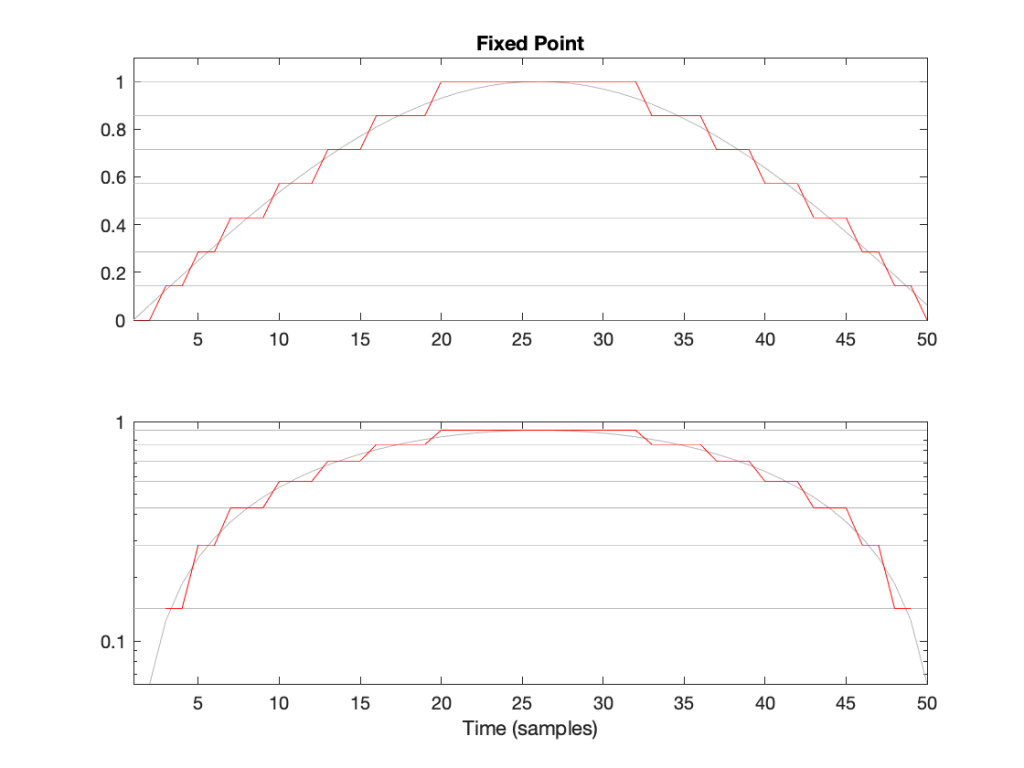Figure 2: The first half of a sine wave (in grey) quantised (without dither) in a simplified 4-bit fixed point system. (I’ve actually cheated a bit and just made 8 equally-spaced steps from 0 to 1 unlike the version shown in Figure 1.) The two plots show identical data, but the bottom plot has a logarithmically-scaled Y-axis.

As can be seen in Figure 2, the equally-spaced steps in a fixed point world mean that the quantisation error is always between -0.5 and 0.5 of a step (a “Least Significant Bit” or LSB), regardless of the level of the signal.

## Floating Point Representation

There is another way to use the bits to represent the signal value. This is to divide the binary “word” into two parts and to do a little math involving some subtraction, multiplication, and an exponent to arrive at the value. Just like in the Fixed Point case, we’ll reserve one bit for the +/- indicator.

Let’s say that we have a 32-bit value to work with. We’ll divide this up into the following:

• 23 bits for the fraction or mantissa, which we’ll abbreviate f
• 8 bits for the exponent, abbreviated e
• 1 bit for the +/- sign (just like in Fixed Point)

We’ll then do the following math:

Sample Value = ± (1 – f) * 2e

We need to know a little extra information:

• because we’re using 23 bits for f, then it can range from 0 to 223-1. In other words, stated mathematically:
0 ≤ 223*f < 223
• because we’re using 8 bits for e, then it has a total range of 28 possible values. In other words it has a range from just over -27 to just under 27. In other words, stated mathematically:
-126 ≤ e ≤ 127
(Note that a couple of possible values are reserved for special purposes, but we won’t talk about those)

This is all a little complicated, but there is a “punch line” to which I’m headed:

Unlike Fixed Point representation, the divisions of the values – the number of steps, and therefore the step sizes – are not the same across the entire scale of possible values. It’s divided into sections, where each section has quantisation steps of equal size, but that step size is dependent on what the value is. In other words the step size changes with the value, but on a coarser scale.

That step size can be calculated as follows:

From 2e to 2e+1, the steps all have an equal size of 2e-fBits where fBits is the number of bits used to express f (in the case of a 32-bit floating point word, fBits = 23 bits). In other words, we have 2fBits equally-spaced steps in that range.

Therefore, each time the signal value moves from just below 0.5 to just above (for example) then the resolution changes, and the higher the value, the lower the resolution. This is is how Floating Point representation behaves.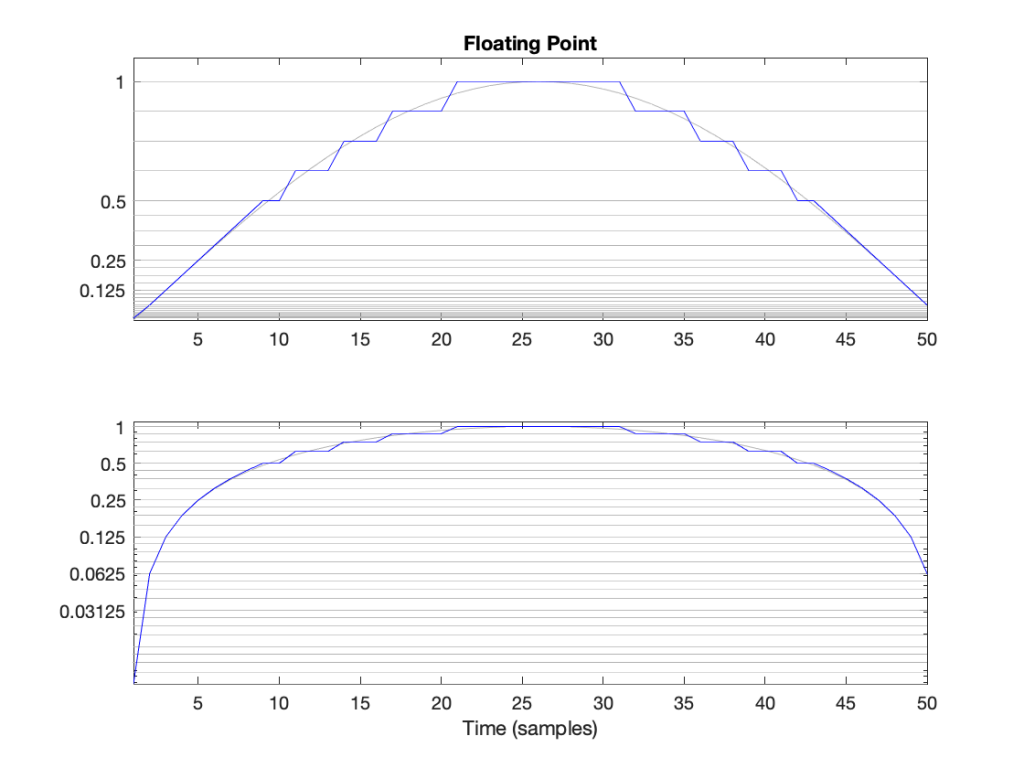Figure 3: The first half of a sine wave (in grey) quantised (without dither) in a simplified floating point system with 2 bits for the fraction. This means that there are 4 equally-spaced steps from (for example) 0.25 to 0.5 or 0.5 to 1. The two plots show identical data, but the top plot has a linearly-scaled Y-axis, whereas the bottom plot has a logarithmically-scaled Y-axis.

## Do I care?

Let’s find out.

In a 32-bit floating point world (therefore, one with a 23-bit fraction), if I have a signal that has a level that has has a maximum positive value of 1 (or 20), then the resolution of the value (which defines the error, which defines the “distance” in dB to the noise floor) is 2-25 (or 1/33,554,432).* This means that the noise floor is about 150 dB below the signal (20 * log10(1 / 2-25). As the signal level drops to 0.5, the noise floor remains the same, so the signal drops by 6 dB, and the SNR reduces to 150 – 6 = 144 dB.

Then, when we drop just below 0.5, the resolution of the value suddenly changes to 2-26 (or 1/67,108,864) , which means that the noise floor is about 150 dB below the signal (20 * log10(0.5 / 2-26). As the signal drops to 0.25 (-6 dB relative to 0.5), the noise floor remains the same, so the signal drops by 6 dB, and the SNR reduces to 150 – 6 = 144 dB.

Then, when we drop just below 0.25, the resolution of the value suddenly changes to 2-27 (or 1/134,217,728), which means that the noise floor is about 150 dB below the signal (20 * log10(0.25 / 2-27). As the signal drops to 0.25 (-6 dB relative to 0.5), the noise floor remains the same, so the signal drops by 6 dB, and the SNR reduces to 150 – 6 = 144 dB.

Hopefully, by now, you’re seeing a pattern here.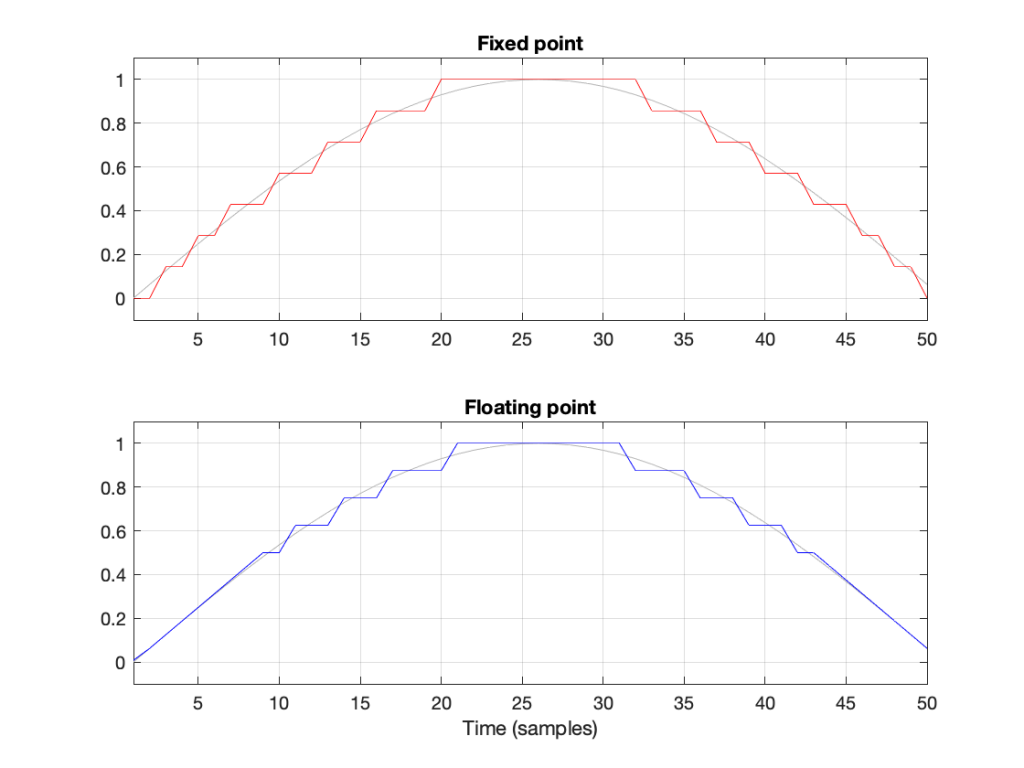Figure 4: Notice that the error of the floating point version is reduced when the signal level (in grey) approaches 0.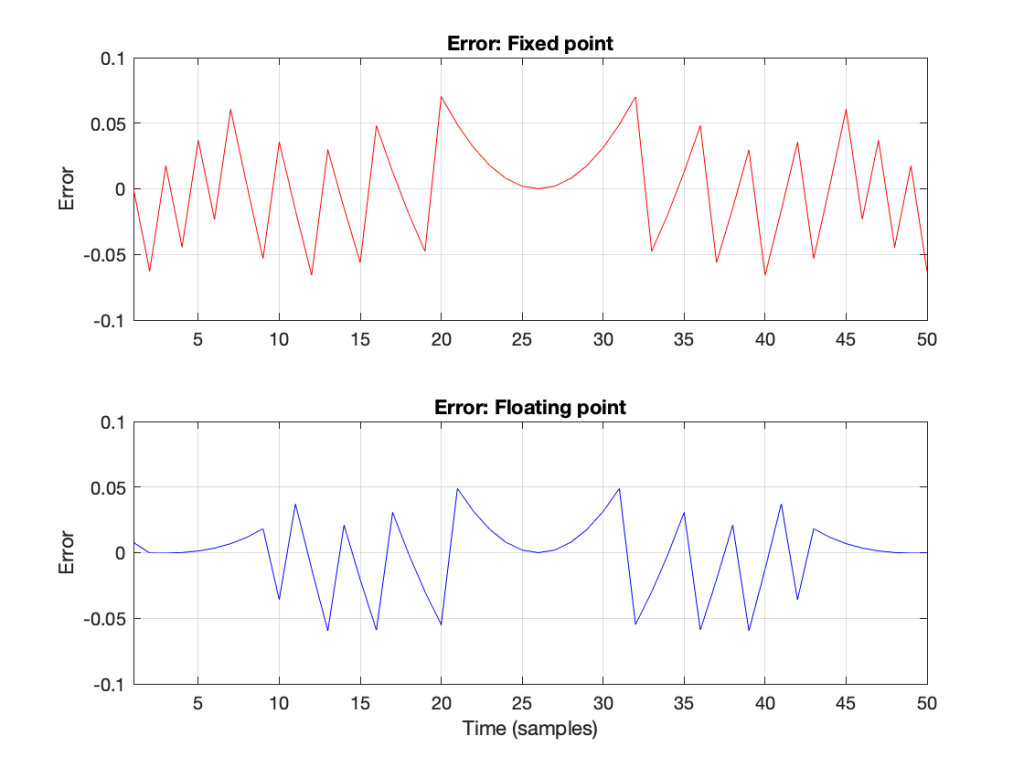Figure 6: The errors from the quantisation shown Figure 5. These are just the original signal subtracted from the quantised signals. Notice that, in Floating Point, the general level of the error is dependent on the level of the signal (it’s smaller on the left and right of the plot) whereas in Fixed Point, the overall level of the error is more constant.

The cool thing is that the pattern would have been the same if I had gone above 1 instead of below it. So, the two things to worry about in Fixed Point (inadequate resolution with (temporarily) low-level signals and clipping when the signal goes outside the range) are not problems in floating point.** And, if you have enough bits (32-bit floating point is the standard “single precision” resolution, but 64-bit “double precision” resolution is not uncommon).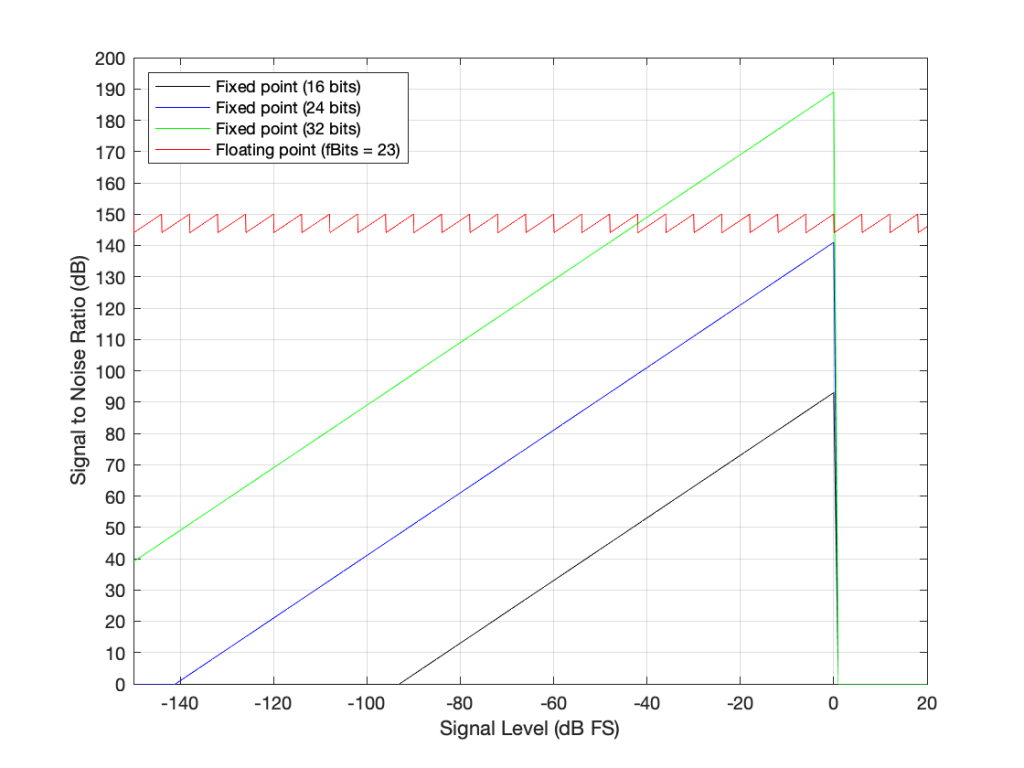Figure 7: The Signal to Distortion+Noise ratio of four different systems, as a function of the signal level in dB FS.**

This is why, in most modern audio systems, you have a fixed-point ADC and a DAC (an Analogue to Digital Converter and a Digital to Analogue converter) at the input and output of your system (because the signal range is reasonably well-defined, and the dynamic range is more than adequate if you do it right) but the processing on the inside is done in 32-bit or 64-bit floating point (or both, in some devices) so that the engineers have the resolution and the range to play with the signals before getting them ready for the output.***

There may be some argument made for a constant noise floor level in a fixed-point system (assuming it’s dithered) over a signal-modulated noise level in a floating-point world (assuming it’s not), however, there are two reasons why this is likely not a real-world issue. The first is that, even in a single-precision floating point system, the worst-case signal to noise ratio is about 144 dB, which is very good. The second is that smart people have already been thinking about dither for floating point systems. If this sounds interesting, you can start reading here

## One last thing

You may be wondering about that sawtooth plot: the red line in Figure 7. It can’t keep going forever, right?

Right.

Eventually, if the signal is quiet enough, then you run out of exponents and the system just behaves as a 23-bit fixed point system (assuming a 32-bit floating point). This will happen when e = -126. Below that, then the SNR just follows a downward slope just like the fixed-point plots. If the signal is loud enough (when e = 127) then you’ll clip, again, just like the fixed-point systems do when the input signal has a level of 0 dB FS.

So, then the question is: “how quiet / loud does the input signal have to be for that to happen?” The answer is very quiet and very loud, as you can see in the plot in Figure 8.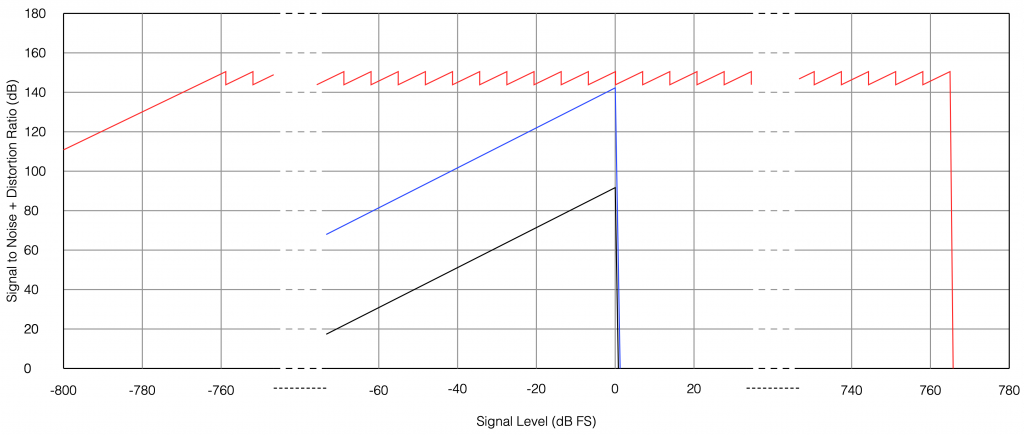Fig 8. The limits of a 32-bit floating point signal. As you can see, you’ve got plenty of dynamic range to work with before you run out of room on either side. The black line is 16-bit fixed point, the blue line is 24-bit fixed point, and the red line is 32-bit floating-point.

You may be wondering how I calculated those limits:

• The first peak in the sawtooth on the left side is at 20*log10(2^-126) = -758.6 dB FS
• The last peak in the sawtooth on the right side is at 20*log10(2^127) = 764.6 dB FS
• The slope that just below the 0 dB FS Signal level is where e = -1. The slope just above 0 dB FS is where e = 0.

## * First small note for the attentive

You may have noticed what appears to be a mistake in my math in there. First I said:

From 2e to 2e+1, the steps all have an equal size of 2e-fBits where fBits is the number of bits used to express f (in our case, fBits = 23 bits). In other words, we have 2fBits equally-spaced steps in that range.

Then I did the math and said

In a 32-bit floating point world (therefore, one with a 23-bit fraction), if I have a signal that has level that has just come up to 1 (or 20), then the resolution of the value (which defines the error, which defines the “distance” in dB to the noise floor) is 2-25 (or 1/133,554,432).

Why did I say 2-25 when maybe I should have said 2-23 (because there are 23 bits in the fraction)? The reason is that the 223 quantisation levels are located between 1 down to 0.5. If I were to continue with the same spacing down to 0, then I would have twice as many quantisation levels, so there would be 224 instead. If I were to continue the spacing all the way down to -1, then there would be twice as many again, or 225.

In other words, a floating point signal ranging from a value of 2-1 to 20 (0.5 to 1) with some number of bits in the fraction that we’re calling fBits will have almost exactly the same signal to noise ratio as an non-dithered fixed point system that is scaled to range from -1 to 1 with fBits+2.

This would be the same from -20 to -2-1 (-1 to -0.5).

At any other signal value, the quantisation behaviours (and therefore the signal-to-noise ratios) of the two systems will be significantly different.

This is visible in Figure 6 where, when the signal is high (in the middle of the plots), the error level is approximately the same in the 4-bit fixed-point system and the floating point system with 2 bits for the fraction.

## ** Second small note for the attentive

You will notice that the black, blue, and green lines in Figure 7 have a sharp transition when the signal level hits 0 dB FS. This is because, in a fixed point system at signal levels below 0 dB FS, the signal to noise ratio is the difference in level between the dither’s noise floor and the signal. The dither level is constant, so as the signal level increases, it gets “further away” from the noise floor until you reach 0 dB FS (with a sine wave), as which point you reach the maximum possible SNR. However, once the signal goes beyond 0 dB FS (still assuming it’s a sine wave), then it starts to clip and distortion components are generated. It does not take much increase in level to drastically increase the level of the distortion relative to the level of the signal (since the signal level cannot increase – you’re just increasing distortion artefacts). Consequently, the signal to distortion+noise drops dramatically, because the distortion components increase in level dramatically.

This does not happen with the floating point system because, at 0 dB FS, you just change the exponent and keep going up with the signal level until you reach the maximum possible exponent value, which goes far beyond what I’ve plotted here.

## Third small note for the attentive

You may be looking at Figure 7 and wondering why the fixed point plots and the floating point plots don’t overlap anywhere. For example, look where the green line (32-bit fixed point) crosses the red line (32-bit floating point). Why don’t they overlap each other there for that little 6 dB-wide range on the X-axis?

The reason is that I’m modelling the fixed point SNRs with TPDF dither, which “costs” 3 dB, but I’m assuming that the floating point signal is not dithered (which would normally be the case). If I were pretending that fixed point didn’t include the dither, then the plots would, indeed, overlap each other for that narrow little window.

## ***One last comment

You may be saying to yourself “But this is nonsense! Why do I need 150 dB SNR when the signal level is lower than -100 dB FS?” The long answer is in this posting, but the short answer is that the signal can go VERY low and VERY high inside a filter (a biquad), so you need to worry about this if you’re doing any changes to the magnitude response of the signal, for example…

Floating Point Numbers posted by Cleve Moler at Mathworks

Floating Point Denormals, Insignificant But Controversial posted by Cleve Moler at Mathworks

# Beolab + 3rd party source with a high-level output

#80 in a series of articles about the technology behind Bang & Olufsen loudspeakers

This week, I was asked a very specific question about connecting an older pair of Beolab loudspeakers to a stereo preamp from another company. Specifically, the owner was wondering why the pairing wasn’t working out too well – and he had already had a theory that the problem had something to do with the sensitivity of his Beolab 9’s.

To be honest, I don’t really know what the problem is with this specific customer’s system – but I made a guess and I figured that the answer might be useful to someone else…

For starters, let’s do some sensitivity training. More accurately, let’s talk about loudspeaker sensitivity. This is a measure of how loud the acoustical output of a loudspeaker is for a given electrical input. Since Beolab loudspeakers are active (meaning, in part, that the amplifiers are built-in) this means that we are talking about an output level in dB SPL for a given input in volts.

For most Beolab loudspeakers, you will get an output of 88 dB SPL for an input of 125 mV RMS if you measure the loudspeaker on-axis in a free field. There are some exceptions to this, most notably Beolab 1, 9, and 5, which will produce 91 dB SPL instead.

So, this tells us how loud the loudspeaker will be for a given input. But my guess is that this had nothing to do with the customer’s problem.

Most customers connect their Beolab loudspeakers to a Bang & Olufsen source using something called a “Power Link” connection. This is a little bundle of wires that contains two audio channels (probably left and right) as well as a data channel (telling the loudspeaker things like the volume setting, for example) and a 5 V DC on/off signal.

Power Link is specified to have a maximum level of 6.5 V RMS, assuming that the signal is a sine wave. This means that a device with a Power Link output can produce no more than 9.2 V Peak. It also means that a device with a Power Link input (like a Beolab loudspeaker) will clip (and therefore distort) at its input if you feed it with more than 9.2 V Peak.

(If you do some math, you can calculate that 20*log10(6.5 V RMS / 125 mV RMS) = 34.3 dB. Therefore, if a Beolab 9 loudspeaker will produce 91 dB SPL with a 125 mV RMS input, then it should produce 91+34.3 dB = 125.3 dB SPL for a maximum accepted input of 6.5 V RMS. Of course, this is not possible – but it’s because the loudspeaker is limited by its drivers, amplifiers, and power supply – not the input maximum input level.)

Back to the question: The customer in question mentioned his stereo preamp’s brand and model number. A little Duck-Duck-Go-ing helped me to find the manual for that particular device, and in the back of that document, I found out that the maximum output level of the preamp was 29 V RMS – which is a lot…

So, the problem is very likely that his preamp is overloading the input stages of the Beolab 9. So, if he turns the volume knob on the preamp up to maximum, and he’s playing a tune that is mastered to be loud on the playback media, then the Beolab 9’s input will be clipped. Changing the sensitivity of the loudspeaker could make it quieter – but it will still be clipped… So the distortion won’t get better – everything will just get quieter.

There are some different solutions to this problem. The easiest one is to not turn up the volume on the preamp – but this is not the best solution, because it means that he’s not using the full dynamic range of the preamp (probably), and therefore that the noise from the preamp is higher in level than it needs to be at the input of the Beolab 9.

There is, however, a very cheap and simple solution, and that is to attenuate the output of the preamp so that when it is set to its maximum output level, it is just hitting the maximum input level of the loudspeaker’s input.

How do we do this? the first question is to find out what the attenuation should be.

Maximum output level = 29.0 V RMS

Maximum input level = 6.5 V RMS

20*log10 (6.5 / 29.0) = -12.99 dB

This is the same as a linear gain of 0.2241.

Now we’re going to build a voltage divider. This is device made of two resistors, placed in series (end-to-end) and connected to the output of the source. The point where the two resistors connect together is used as the output to the loudspeaker, resulting in a schematic as shown below.

As you can probably see in the schematic, the grounds of the two devices (which are connected to the exterior casings of the RCA Phono plugs) are connected together. As the voltage on the pin of the source goes up and down, the voltage on the pin of the loudspeaker also goes up and down – but by less. How much less is determined by the values of the resistors.

For example, if the resistors are equal (R1 = R2) then the output will be half of the input. If R2 is one tenth of the total of R1+R2, then the output will be one tenth of the input. You can calculate this gain yourself with a simple equation:

Linear Gain = R2 / (R1 + R2)

and

Gain in dB = 20 * log10 (Linear Gain)

So, for example, if R1 = 8,000 Ω and R2 = 2,000 Ω, then the gain will be

2000 / (8000 + 2000) = 0.2

which is equal to 20*log10 (0.2) -13.98 dB.

Unfortunately, if you want to do this with only two resistors, you can’t be too choosy about their resistances. There are standard resistor values, and you’ll have to pick from that list.

Also, it’s a good “rule of thumb” to try and keep the resistance “seen” by the source around 10,000 Ω (or 10 kΩ) – just to keep it happy. If you make the value too low, then you will be asking for it to deliver too much current (and its maximum output level will drop). If you make it too high, you might create and antenna and result in some extra noise…

So, I want to make R1 +R2 about 10,000 Ω, and I want R2 / (R1+R2) to be about 0.2241 (because I’m trying to convert 29 V RMS to 6.5 V RMS). So, I go to a list of standard resistor values like this one and I start trying to simultaneously fulfill those two requirements.

After some trial and error, I find out that if I make R1 = 8.2 kΩ and R2 = 2.4 kΩ, I can come pretty close.

2400 / (8200 + 2400) = 0.2264 = -12.902

close enough. Now I just need to get a soldering iron and a bit of wire, and put it all together…

## The details…

However, if you clicked on that list of standard resistor values, you might notice that it says ±5% at the top of the table. This is normal. If you go to your local resistor store and you buy a 1 kΩ resistor – it probably won’t be exactly 1,000.000000000 Ω. But it will be close. If you buy from the ±5% stack, then any resistor in that bunch will be within 5% of the stated value. So, for a 1 kΩ resistor ±5%, it will be somewhere between 950 Ω and 1050 Ω.

So, then the question is, for the resistors that I just picked, how bad can it get, and is that good enough?

Well… this can be calculated. I just put in the worst-case values for my two resistors into the math, and do it over and over until I get all the possible answers. This would look like this:

If we look at this in terms of how far away we are from the target – the gain error, then it looks like this:

So, if we randomly choose resistors out of the bag, the worst that can happen is that we will be 0.6 dB below the target or 0.8 dB above the target.

This means that, if we’re not careful, and we’re unlucky, then we can get a mismatch between the two channels of 1.4 dB (assuming that one channel was a worst-case low and the other is a worst-case high). This is enough to be audible as about a 15% shift towards the louder loudspeaker, which is probably not acceptable.

So, the moral of the story is that you should measure your resistors before soldering them into the circuit.

Note, however, that it’s not necessary to make the gains perfect to improve the imaging. You just need to make them equal in the two channels for that…

## Speaking Passively

The circuit I show above is called a “passive” circuit. This means that it doesn’t require any external power source (like a battery or a power supply) to work. However, it also means that it can’t make things louder – no matter what resistor values you choose, the output will always be less than the input.

There are lots of reasons why this is a useful little circuit. It’s cheap, it’s easy to make, it’s small (you could hide it inside one of the RCA connectors), and it will prevent you from overloading the input of the downstream device (in this case, a loudspeaker). Not only that, but it will also attenuate the noise generated by the source – so not only will the customer’s system no longer clip, it will also (probably) have a lower noise floor.

# Resolution, Part 1: White Noise

This is the start of what will be a series of posts that are an attempt to answer a question about the pro’s and con’s of implementing a volume control in the digital domain. When I first thought about how to answer this question, I thought I could do it in a couple of sentences – but the more I thought about it, the more I realised that the answer is complicated…

There’s no doubt in my mind that I’m making this answer more complicated than necessary, but, as Carl Sagan once said, “If you wish to make apple pie from scratch, you must first create the universe.”

So, to begin, we have to define what “noise” is from the point of view of audio engineering.

On the one hand, we can define it simply. “Noise” is a random signal. We can be more accurate and say that this means that the amplitude of a noise signal cannot be predicted using a knowledge of what has come before in time.

If I flip a coin, it will be either heads or tails. I can’t predict this. It will be random. If I flip it 100 times, and, by some strange coincidence, I get 100 “tails”, there is still a 50% chance of getting a tails on the 101st flip. What has happened before can, in no way, be used to predict what is about to happen.

Of course, what is about to happen on the 101st flip has a limited number of possible outcomes. I cannot flip the coin and get “dog” as a result… (this sounds silly, but it will come in handy later…) Just like I cannot roll two dice and get a 13…

In LPCM digital audio, a noise signal is one where each individual sample in the signal has a random value that is in no way related to any of the previous samples. Its range (the set of possible values from which we can pick our random number) may be limited (depending on the specific characteristics of the noise signal and what may have come before), but it will be random.

Typically, when you are talking to someone in audio about noise, they describe it using a colour as the first descriptor. So, you’ll hear of “white noise” and “pink noise”, as the two most popular examples. For the purposes of this series of postings, we’ll only be talking about white noise. So, what is this?

One definition that you’ll see thrown around a lot says something like “white noise is a random signal that has equal energy per linear bandwidth” or “… equal energy per hertz” or “…equal intensity at different frequencies” or something like this. These descriptions are sort of true if you don’t want to get into temporal details, which, unfortunately, is exactly where we’re headed…

The good thing about those definitions is that they describe a general characteristic of white noise. If you take a white noise signal, and you measure the intensity of (or the energy in) the signal for a given bandwidth (say, a bandwidth of 100 Hz ranging from 200 Hz to 300 Hz) then it will be the same in another frequency range with the same bandwidth (say, a bandwidth of 100 Hz ranging from 1,000 Hz to 1,100 Hz). Note that these two bandwidths are the same in hertz – not in a multiplier like octaves or semitones or decades. So, if you have white noise that has a total bandwidth of 0 Hz to 20,000 Hz, then you will have the same amount of energy in the 0 – to – 10,000 Hz band as you will in the 10,000 – to – 20,000 Hz band. In other words (to us humans), there is as much energy in the top octave of the signal as in the rest of the bandwidth combined.

This is why white noise sounds like “bright” and “hissy” (similar to the “ss” sound in “hissy”) and not “darker” like the “sh” sound in “ash” (as they incorrectly claim here…). Since white is a “bright” colour, then we use the word “white” to describe the frequency-dependent energy distribution of “white” noise.

However, this is not really true. The truth is that a white noise signal has an equal probability per bandwidth of having the same energy level. This little detail is usually left out, partly because it’s complicated, and partly because it doesn’t matter in most cases in the real world. However, in our case, it does.

Let’s look at an example. I made a white noise signal in Matlab using the statement
rand(SignalLength, 1) – rand(SignalLength, 1)
where SignalLength is the length of the noise signal in samples, and the 1 means that I’m doing this for 1 audio channel…. mono is so retro…

You may be wondering why I did a
rand() – rand()
rand().
the simple answer for now was that I wanted to make the signal “balanced” on either side of the zero line and the rand() function in Matlab has a range of 0 to 1.

I know… I could have done this by saying
2 * (rand(SignalLength, 1) – 0.5)
but there is another reason that we’ll get into later…)

I then used a DFT to find the magnitude response of this signal. The result – both the signal and its magnitude response – are shown below in Figure 1.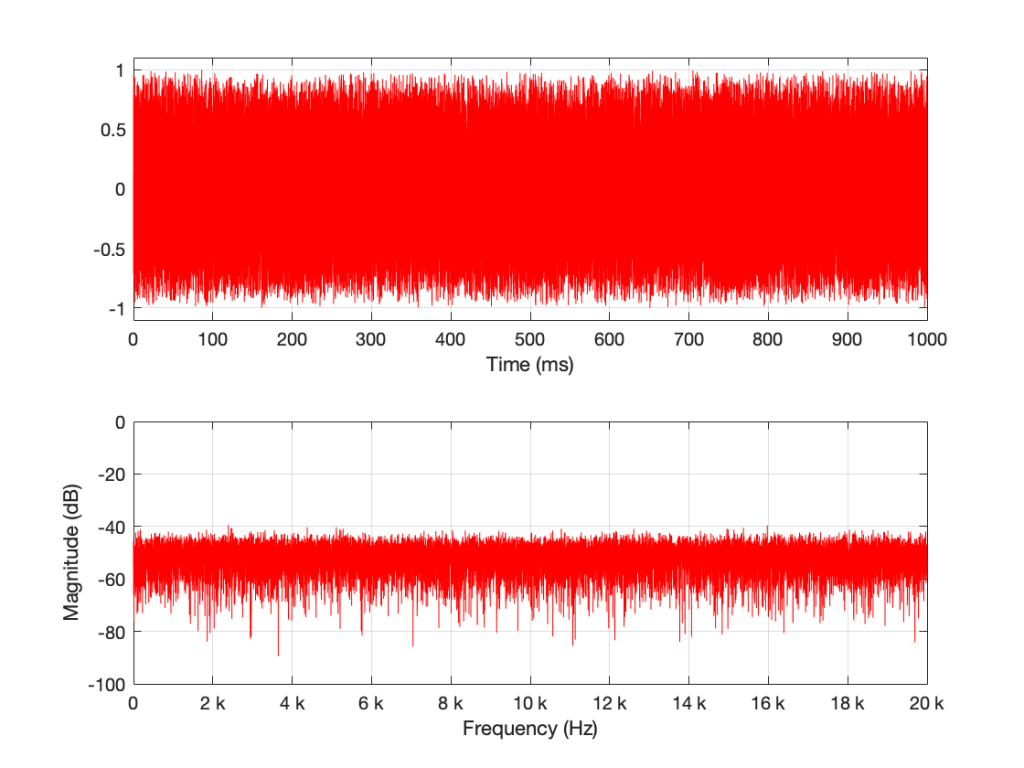Figure 1: A random signal shown in the time domain (top plot) and its magnitude response (bottom plot).

Some additional information that is really not important: The sampling rate of this signal is 2^16 (65,536 Hz), and I did a 2^16 point DFT, so I have one frequency bin per hertz. (If this last bit of information is confusing, but interesting, please start reading this…)

You may notice that the magnitude is “flat” – meaning that it generally doesn’t slope upwards or downwards. However, you will also notice that it is certainly not “flat” – meaning that it is not a perfectly straight line. In fact, if we zoom in on both plots, we can see Figure 2.

Notice that we do NOT have an equal amount of energy per hertz… if we did, then the bottom plot would be a flat line.

If I do all of that again – make a new noise sample the same way (with a new set of random numbers) and plot the result, and a zoomed in version, I get Figures 3 and 4.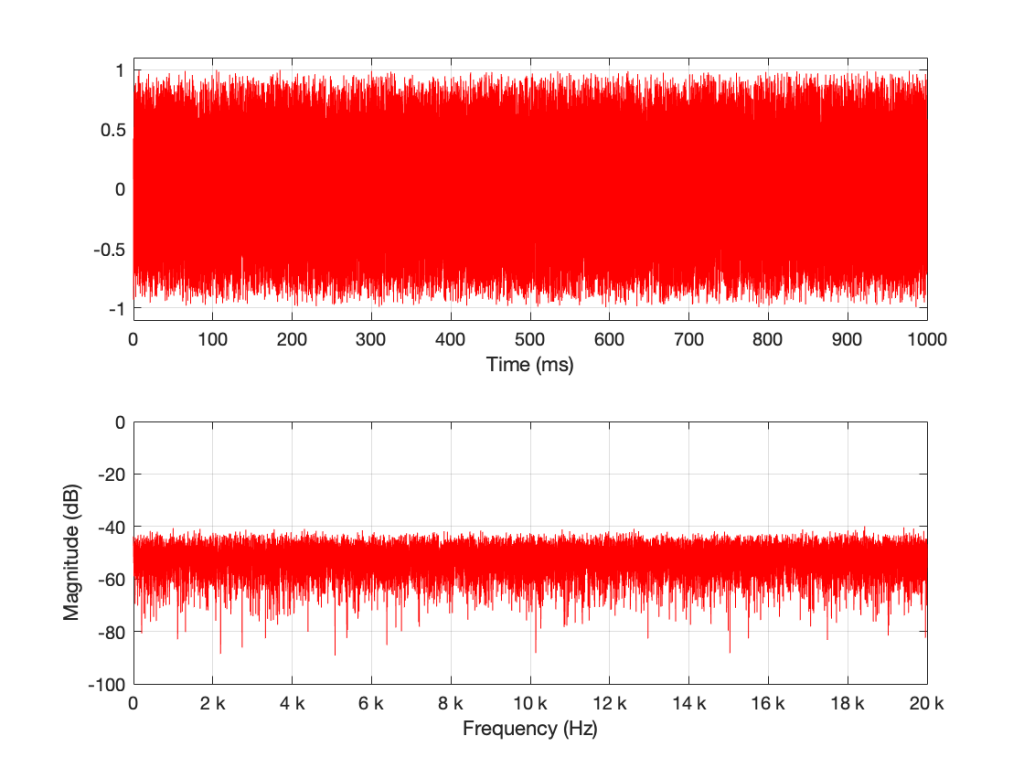Figure 3: The result of running the same code that generated Figure 1. However, this is a new set of random numbers. Notice that, on first glance, it is the same as Figure 1, but if you look carefully, it is completely different.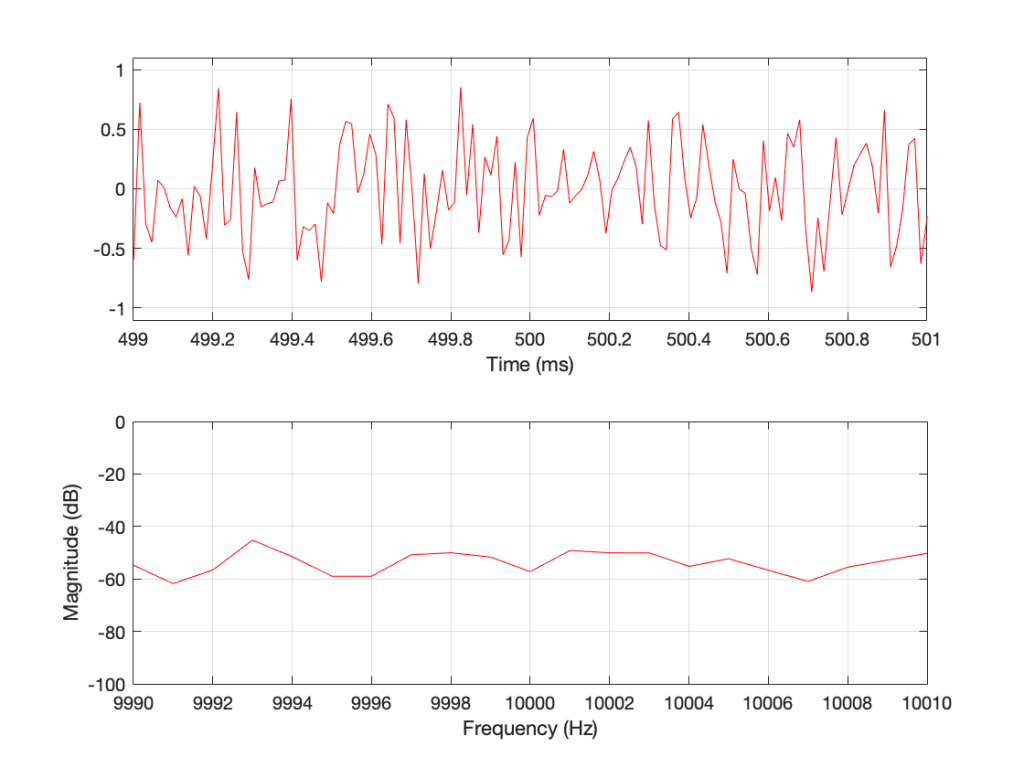Figure 4: A detail from Figure 3. Notice that, on first glance, it is the same as Figure 2, but if you look carefully, it is completely different.

Compare Figures 1 and 3 or Figure 2 and 4. You’ll notice that they have similar characteristics overall – but not only are they NOT identical, they are completely different (on a sample-by-sample or a DFT bin-by-bin comparison).

Let’s say that I run this code and generate a white noise signal 1 second long, and I then calculate the magnitude response of that noise signal and store it. Then, I’ll repeat this, and average the new magnitude response to the first one. Then, I’ll do it again, each time, including the magnitude response to the average of all of the magnitude responses that I’ve done….

For each 1-second slice of time, the noise signal does not have equal energy per bandwidth – however, it is certainly white noise.

This is because, each time I do this, the average magnitude response will get flatter and flatter… and eventually, after doing this an infinite number of times, it will be a flat line.

This means that, white noise will have an equal amount of energy per bandwidth only if I wait long enough. The question is “how long is long enough?” The answer to that question depends on what you’re doing with it.

## Another way to look at this…

In the each of the examples above, I made 1 second-long white noise signals and used the entire signal – all 65,536 samples – to calculate the magnitude response.

What happens if I have a one-second long signal, but only a portion of it is a burst of white noise, and the rest is silence? For example, look at Figure 5.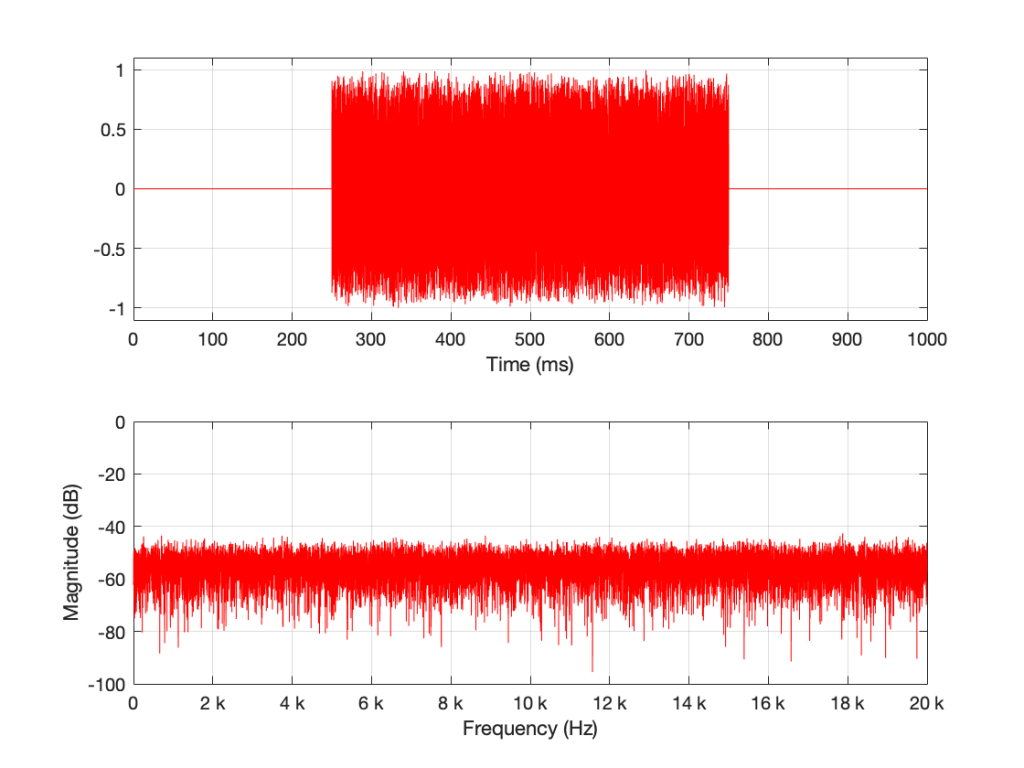Figure 5: A 1-second long signal that contains a burst of white noise for 0.5 seconds.

Figure 5’s magnitude response looks similar to the ones we’ve seen before (apart from being a little lower overall than the plots in Figures 1 and 3 – because there’s less energy overall in 0.5 sec of noise than there is in 1 second of noise). I’ll keep going to show what happens if we take this to an extreme.

The magnitude response shown in Figure 7 looks very different from the ones we’ve seen before. It’s much smoother… We’ll keep going…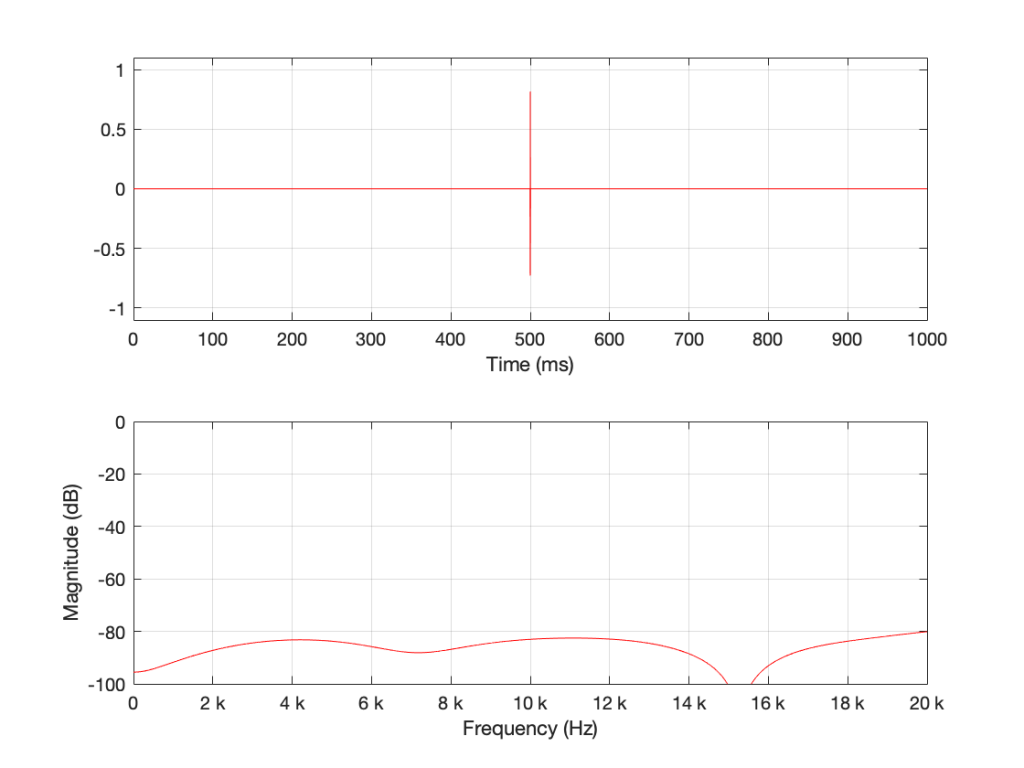Figure 8. 16 samples of white noise in the middle of a 65,536 sample long signal of silence. Notice that, even when not “zoomed in”, the magnitude response is smooth

Figure 8 is very different again… The total magnitude response, even when not “zoomed in” is smooth. It’s important here to note that the actual response that we see there will be different every time I run the random generator again. For example, look at Figure 9, which is also a 16-sample long white noise signal.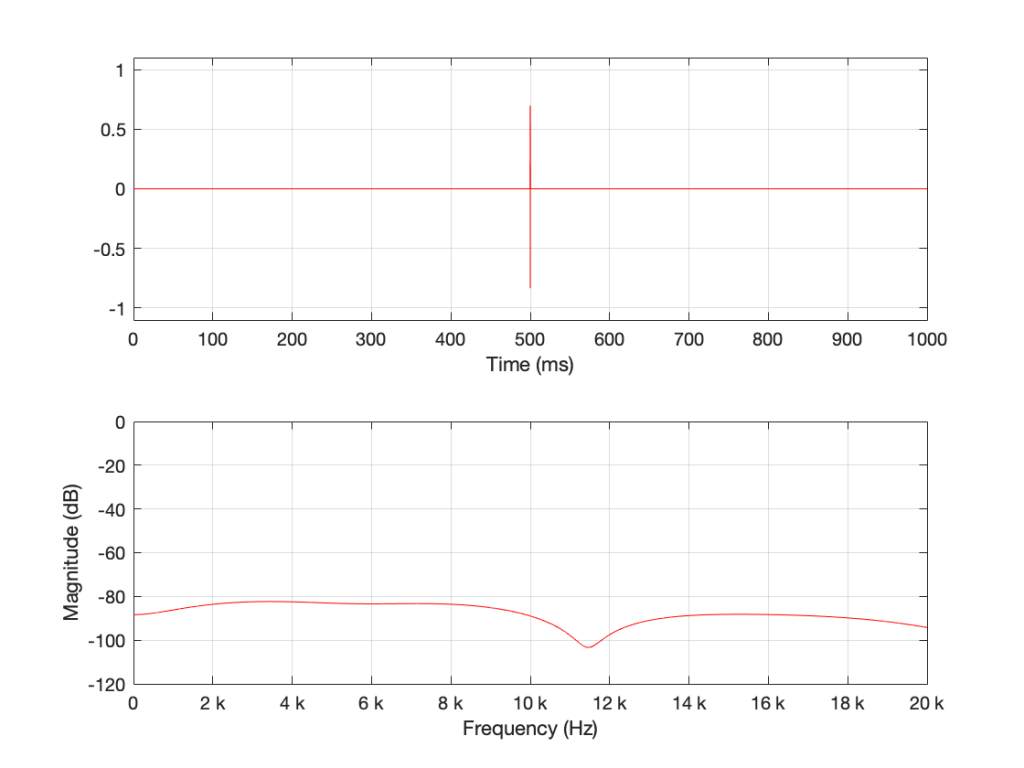Figure 9. 16 samples of white noise in the middle of a 65,536 sample long signal of silence. Notice that, even when not “zoomed in”, the magnitude response is smooth – but it is not identical to the one in Figure 8 because the random numbers are not the same.

If we keep getting shorter and shorter, eventually we’ll get down to a single sample with a random value. However, since it’s a single sample (that is very probably non-zero) in a long string of zeros, then its magnitude response will be completely flat. It will not be noise – it will be an impulse with a random level. And it won’t sound like noise – it will sound like a click.

Summary

There are two basic important things to know at this point.

• White noise has the frequency content you expect only if you average over time.
• The shorter the time the noise is present, the less energy you will have, overall.

The discussion continues in Part 2.

## P.S.

Thanks to David for emailing and pointing out that it’s “Hz” and “hertz” but not “Hertz”. I’ve corrected the text above… Being reminded of this reminds me of a Steven Wright joke – “I’m having amnesia and déjà vu at the same time. I think I’ve forgotten this before…”